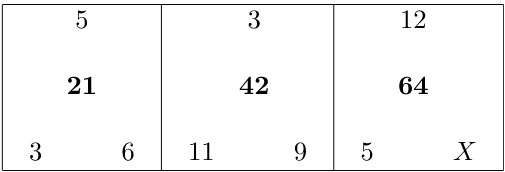Chapter 2: Linear Equations

# 2.8 The Mystery X Puzzle

The centre number of each square is found by using the order of operations on the numbers that surround it. The challenge is to solve for the variable $X.$Can you solve for $X$? Can you find any other possible solution?